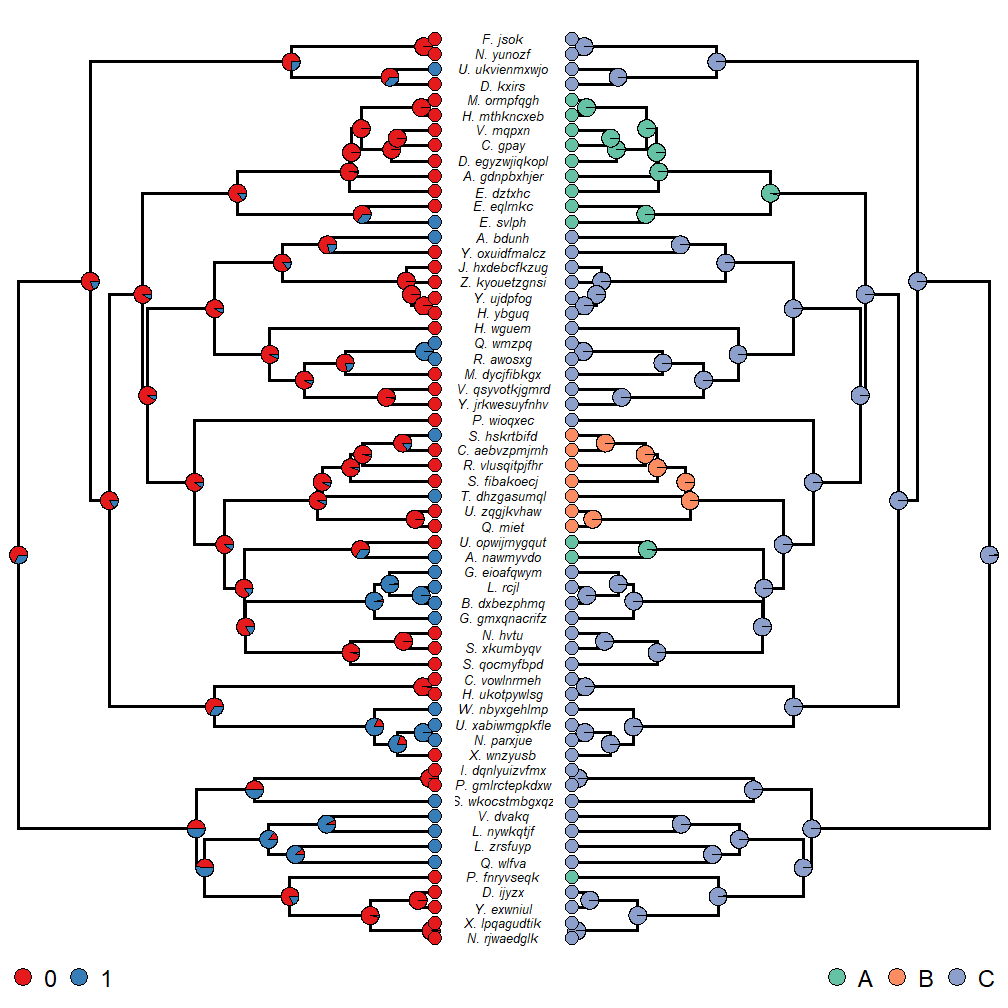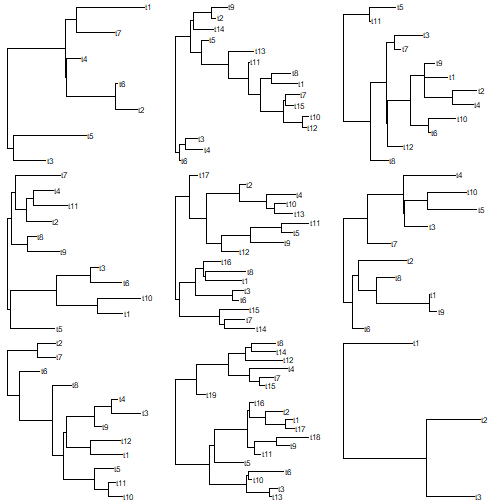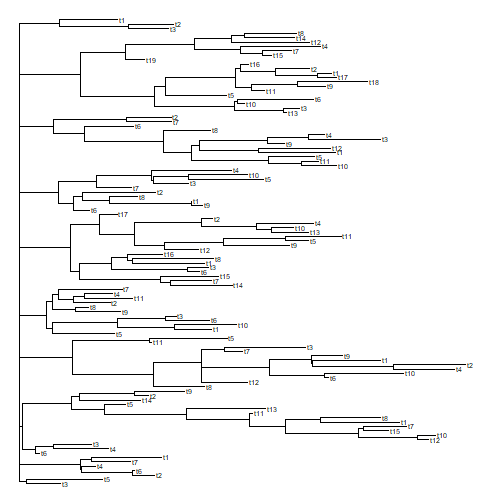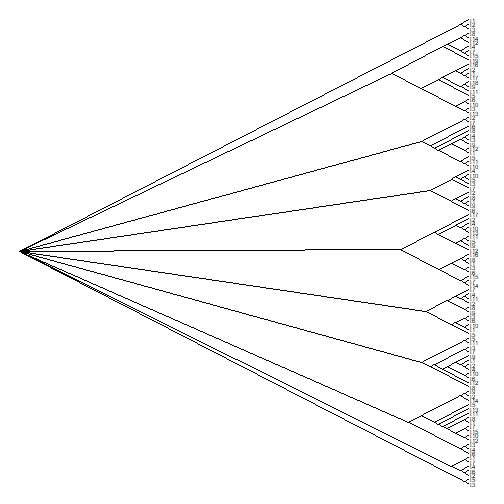## Wednesday, February 21, 2018

### A refresher on plotting facing phylogenies with node labels

An R phylogenetics user today contacted me about plotting two identical facing trees, along with reconstructed ancestral states at internal nodes, but with only one set of tip labels between them.

This is easy enough to do, so let's see how.

First our tree & data are simulated - but with realistic looking tip labels.

``````library(phytools)
library(RColorBrewer)
cw<-untangle(tree,"read.tree") ## make sure tips are in order
layout(matrix(1:3,1,3),widths=c(0.45,0.1,0.45))
plotTree(cw,ftype="off",ylim=c(-1,Ntip(cw)))
obj<-rerootingMethod(cw,x,model="ER")
k<-length(levels(x))
cols<-setNames(brewer.pal(max(c(3,k)),"Set1")[1:k],levels(x))
nodelabels(pie=obj\$marginal.anc,piecol=cols,cex=1)
tiplabels(pie=to.matrix(x,levels(x)),piecol=cols,cex=0.8)
legend(x="bottomleft",legend=levels(x),pch=21,pt.cex=2.5,pt.bg=cols,cex=1.5,
bty="n",horiz=T)
ylim<-get("last_plot.phylo",envir=.PlotPhyloEnv)\$y.lim
plot.new(); plot.window(xlim=c(-0.1,0.1),ylim=ylim)
text(rep(0,Ntip(cw)),1:Ntip(cw),gsub("_"," ",cw\$tip.label),cex=0.8,
font=3)
plotTree(cw,ftype="off",ylim=c(-1,Ntip(cw)),direction="leftwards")
obj<-rerootingMethod(cw,y,model="ER")
k<-length(levels(y))
cols<-setNames(brewer.pal(max(c(3,k)),"Set2")[1:k],levels(y))
nodelabels(pie=obj\$marginal.anc,piecol=cols,cex=1)
tiplabels(pie=to.matrix(y,levels(y)),piecol=cols,cex=0.8)
legend(x="bottomright",legend=levels(y),pch=21,pt.cex=2.5,pt.bg=cols,cex=1.5,
bty="n",horiz=T)
``````Of course, some of the plotting parameters - such as the font size, line width, and the sizes of the plotted pies - will need to be adjusted depending on the size of our tree; but hopefully this is a good starting point!

FYI, here's how the tree & data were simulated:

``````N<-60
tips<-paste(sample(LETTERS,N,replace=T),"._",
replicate(60,paste(sample(letters,sample(4:12,1)),
collapse="")),sep="")
tree<-pbtree(n=N,tip.label=tips,scale=1)
Q1<-matrix(c(-1,1,1,-1),2,2,dimnames=list(0:1,0:1))
x<-sim.Mk(tree,Q1)
Q2<-matrix(c(-0.4,0.2,0.2,0.2,-0.4,0.2,0.2,0.2,-0.4),3,3,
dimnames=list(LETTERS[1:3],LETTERS[1:3]))
y<-sim.Mk(tree,Q2)
``````

## Tuesday, February 20, 2018

### Recursive `bind.tree` function

A phytools user contacted me today about coding a recursive version of `ape::bind.tree` - that is, a function that would take a list of trees & bind them successively together (based on his code, at the root) until only one tree remained.

A recursive function is nothing more than a function that calls itself internally. These are possible, though (in my experience) not that common, in R.

I'm not sure why we'd want to do this since a simple `for` or `while` loop could do the same thing, but I thought I'd help him out anyway.

Here's my recursive function:

``````bind.trees<-function(trees){
if(length(trees)==2) return(bind.tree(trees[],trees[]))
else {
trees<-c(bind.tree(trees[],trees[]),
if(length(trees)>2) trees[3:length(trees)])
trees<-bind.trees(trees) ## this is the recursive part
return(trees)
}
}
``````

We can test it out as follows:

``````trees<-lapply(sample(2:20,9,replace=TRUE),rtree)
trees<-lapply(trees,function(x){
x\$root.edge<-runif(n=1)
x})
class(trees)<-"multiPhylo"
print(trees,details=TRUE)
``````
``````## 9 phylogenetic trees
## tree 1 : 7 tips
## tree 2 : 15 tips
## tree 3 : 12 tips
## tree 4 : 11 tips
## tree 5 : 17 tips
## tree 6 : 10 tips
## tree 7 : 12 tips
## tree 8 : 19 tips
## tree 9 : 3 tips
``````
``````par(mfrow=c(3,3))
plotTree(trees,lwd=1)
````````````tree<-bind.trees(trees)
plotTree(tree,fsize=0.6,lwd=1)
``````Or, to better see the structure of all our original trees:

``````tree\$edge.length<-NULL